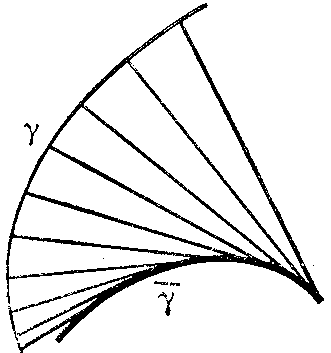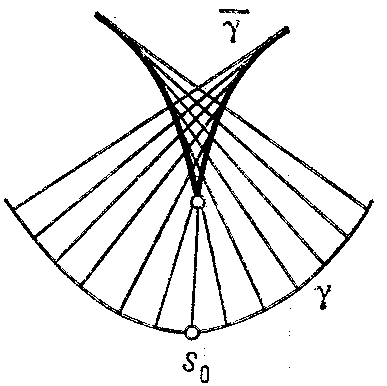# Evolute

of a plane curve

The set $\widetilde \gamma$ of the centres of curvature of the given curve $\gamma$. If $\mathbf r = \mathbf r ( s)$( where $s$ is the arc length parameter of $\gamma$) is the equation of $\gamma$, then the equation of its evolute has the form

$$\widetilde{\mathbf r} = \mathbf r + \frac{1}{k} {\pmb\nu } ,$$

where $k$ is the curvature and ${\pmb\nu }$ the unit normal to $\gamma$. The figures shows the construction of the evolute in three typical cases:

a) if along the entire curve $k ^ \prime$ has a fixed sign and $k$ does not vanish;Figure: e036670aFigure: e036670b

b) if along the entire curve $k ^ \prime$ has a fixed sign and $k$ vanishes for $s = s _ {0}$; and

c) if $k ^ \prime > 0$ for $s < s _ {0}$; $k ^ \prime ( s) < 0$ for $s > s _ {0}$; $k ^ \prime ( s _ {0} ) = 0$, and $k$ does not vanish (the point of the evolute corresponding to $s = s _ {0}$ is a cusp).Figure: e036670c

The length of the arc of the evolute corresponding to the segment $s _ {1} \leq s \leq s _ {2}$ of $\gamma$ is

$$\widetilde{s} ( s _ {1} , s _ {2} ) = \left | \frac{1}{k ( s _ {2} ) } - \frac{1}{k ( s _ {1} ) } \right | .$$

The evolute is the envelope of the normals to $\gamma$. The curve $\gamma$ is called the evolvent of its evolute (cf. Evolvent of a plane curve).

The evolvent is also called the involute; thus, if $\gamma ^ \prime$ is the evolute of $\gamma$, then $\gamma$ is the involute of $\gamma ^ \prime$, cf. Evolvent of a plane curve.